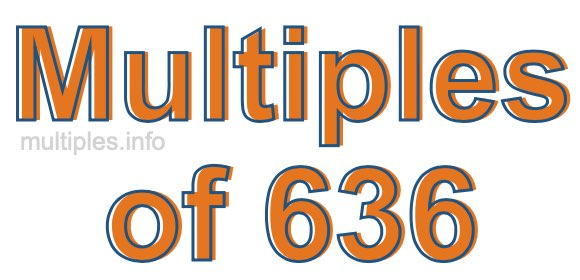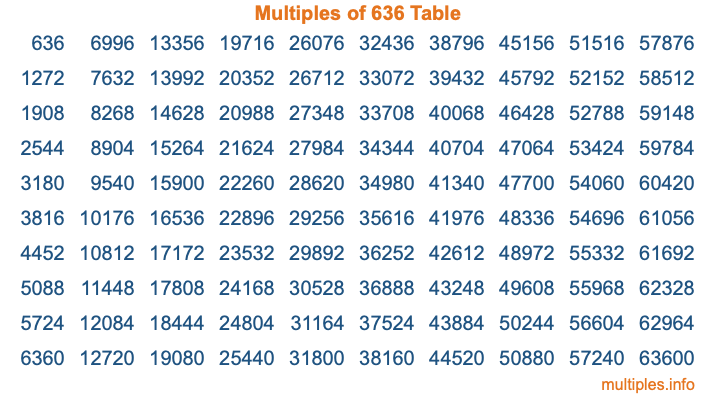Multiples of 636Welcome to the Multiples of 636 page. Here we will first teach you everything you will ever need to know about the multiples of 636, and then give you a study guide summary of everything we taught you to make sure you remember it all. Use this page to look up facts and learn information about the multiples of 636. This page will make you a multiples of six hundred thirty-six expert!

Definition of Multiples of 636
Multiples of 636 are all the numbers that when divided by 636 equal an integer. Each of the multiples of 636 are called a multiple. A multiple of 636 is created by multiplying 636 by an integer.

Therefore, to create a list of multiples of 636, you start with 1 multiplied by 636, then 2 multiplied by 636, then 3 multiplied by 636, and so on for as long as you want. Thus, the list of the first five multiples of 636 is 636, 1272, 1908, 2544, and 3180. To see a larger list of multiples of 636, see the printable image of Multiples of 636 further down on this page. We also have a category where you can choose any nth multiple of 636.

Multiples of 636 Checker
The Multiples of 636 Checker below checks to see if any number of your choice is a multiple of 636. In other words, it checks to see if there is any number (integer) that when multiplied by 636 will equal your number. To do that, we divide your number by 636. If the the quotient is an integer, then your number is a multiple of 636.

Is  a multiple of 636?

Least Common Multiple of 636 and ...
A Least Common Multiple (LCM) is the lowest multiple that two or more numbers have in common. This is also called the smallest common multiple or lowest common multiple and is useful to know when you are adding our subtracting fractions. Enter one or more numbers below (636 is already entered) to find the LCM.

Check out our LCM Calculator if you need more details about the Least Common Multiple or if you need the LCM for different numbers for adding and subtraction fractions.

nth Multiple of 636
As we stated above, 636 is the first multiple of 636, 1272 is the second multiple of 636, 1908 is the third multiple of 636, and so on. Enter a number below to find the nth multiple of 636.

th multiple of 636

Multiples of 636 vs Factors of 636
636 is a multiple of 636 and a factor of 636, but that is where the similarities end. All postive multiples of 636 are 636 or greater than 636. All positive factors of 636 are 636 or less than 636.

Below is the beginning list of multiples of 636 and the factors of 636 so you can compare:

Multiples of 636: 636, 1272, 1908, 2544, 3180, etc.

Factors of 636: 1, 2, 3, 4, 6, 12, 53, 106, 159, 212, 318, 636

As you can see, the multiples of 636 are all the numbers that you can divide by 636 to get a whole number. The factors of 636, on the other hand, are all the whole numbers that you can multiply by another whole number to get 636.

It's also interesting to note that if a number (x) is a factor of 636, then 636 will also be a multiple of that number (x).

Multiples of 636 vs Divisors of 636
The divisors of 636 are all the integers that 636 can be divided by evenly. Below is a list of the divisors of 636.

Divisors of 636: 1, 2, 3, 4, 6, 12, 53, 106, 159, 212, 318, 636

The interesting thing to note here is that if you take any multiple of 636 and divide it by a divisor of 636, you will see that the quotient is an integer.

Multiples of 636 Table
Below is an image of the first 100 multiples of 636 in a table. The table is in chronological order, column by column. The first column has the first ten multiples of 636, the second column has the next ten multiples of 636, and so on.The Multiples of 636 Table is also referred to as the 636 Times Table or Times Table of 636. You are welcome to print out our table for your studies.

Negative Multiples of 636
Although not often discussed or needed in math, it is worth mentioning that you can make a list of negative multiples of 636 by multiplying 636 by -1, then by -2, then by -3, and so on, to get the following list of negative multiples of 636:

-636, -1272, -1908, -2544, -3180, etc.

Multiples of 636 Summary
Below is a summary of important Multiples of 636 facts that we have discussed on this page. To retain the knowledge on this page, we recommend that you read through the summary and explain to yourself or a study partner why they hold true.

There are an infinite number of multiples of 636.

A multiple of 636 divided by 636 will equal a whole number.

636 divided by a factor of 636 equals a divisor of 636.

The nth multiple of 636 is n times 636.

The largest factor of 636 is equal to the first positive multiple of 636.

636 is a multiple of every factor of 636.

636 is a multiple of 636.

A multiple of 636 divided by a divisor of 636 equals an integer.

636 divided by a divisor of 636 equals a factor of 636.

Any integer times 636 will equal a multiple of 636.

Multiples of a Number
Here you can get the multiples of another number, all with the same attention to detail as we did for multiples of 636 on this page.

Multiples of
Multiples of 637
Did you find our page about multiples of six hundred thirty-six educational? Do you want more knowledge? Check out the multiples of the next number on our list!